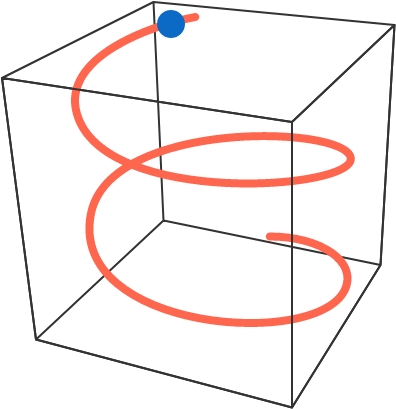# Sliding Down a Helix

A bead slides from rest down a wire that's bent into a helix, which can be parametrized in the following way: $\begin{cases} x = \cos(\theta) \\ y = \sin(\theta) \\ z = \theta. \end{cases}$ Find the magnitude of the bead's vertical acceleration.Assumptions: The bead slides without friction and there is a uniform, gravitational field $-g\,\hat{\mathbf{z}}.$

×